# Chappuis Halder & Co - VaR estimation solutions

Jun. 30, 2015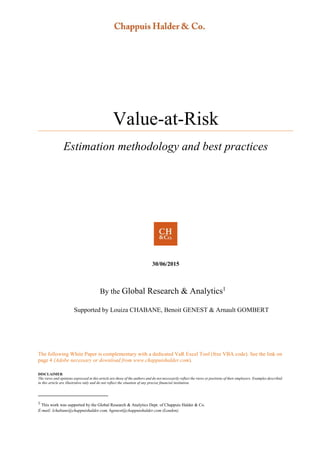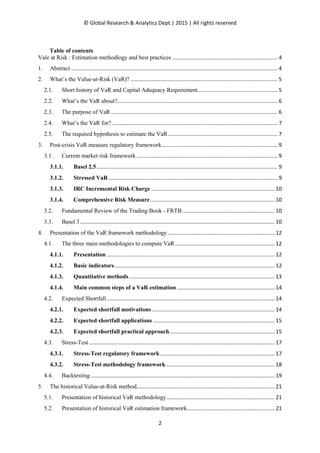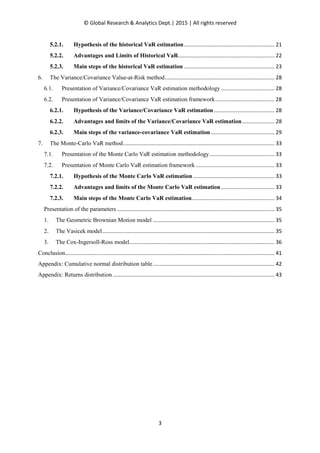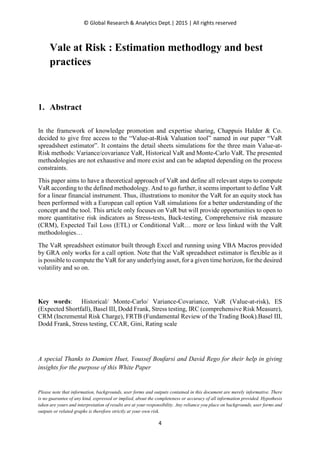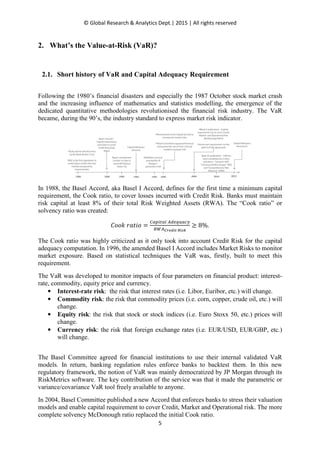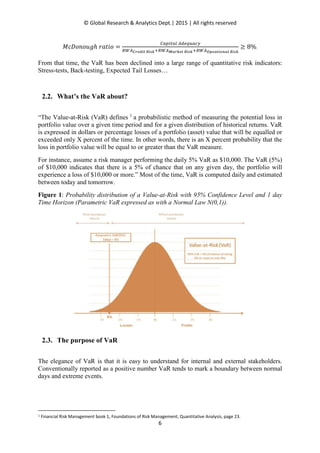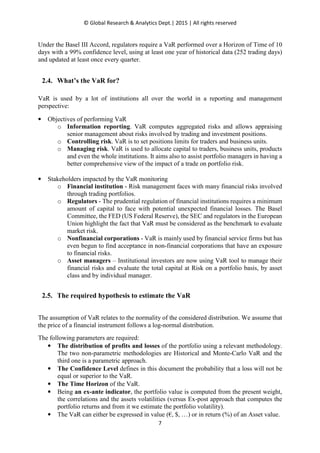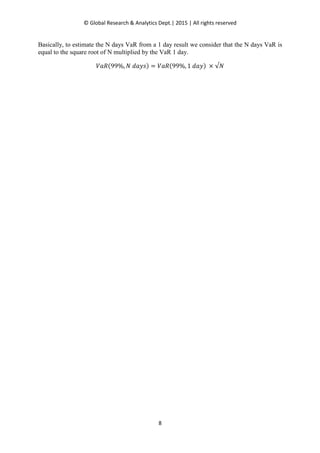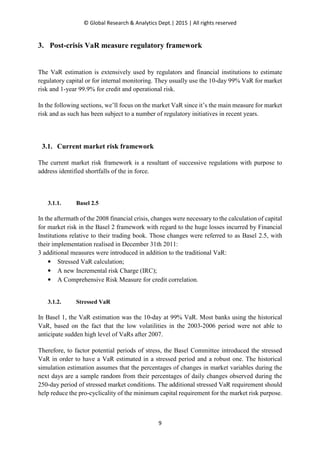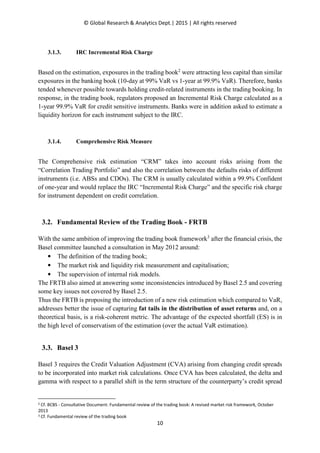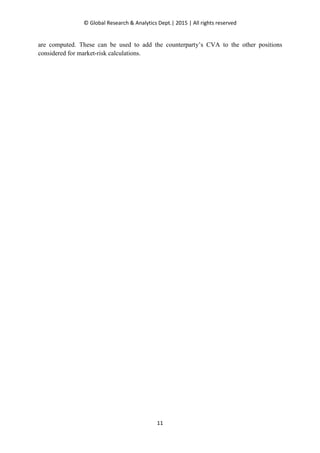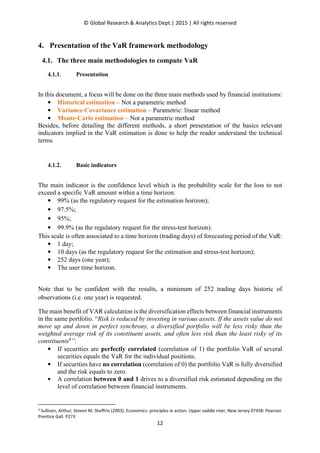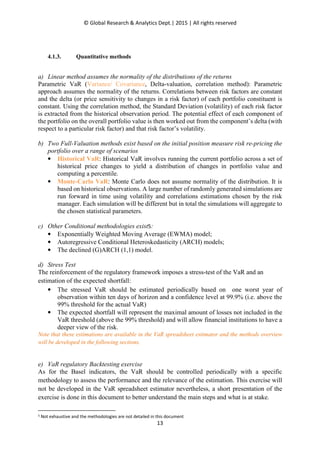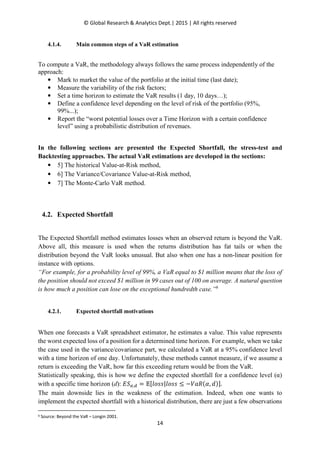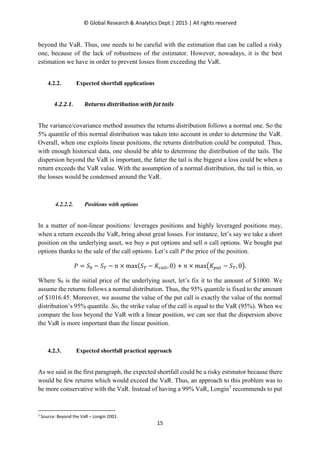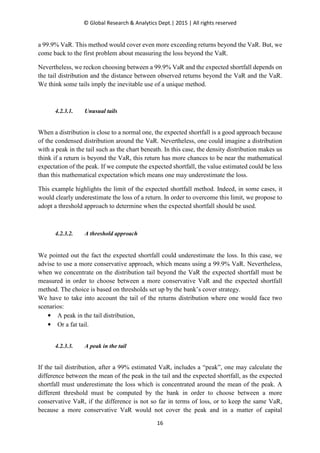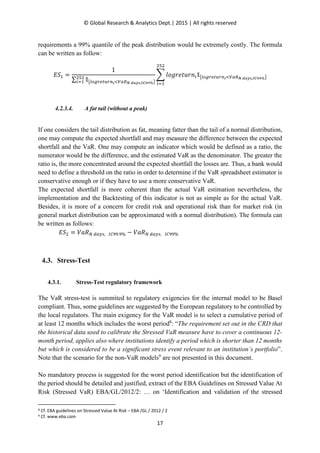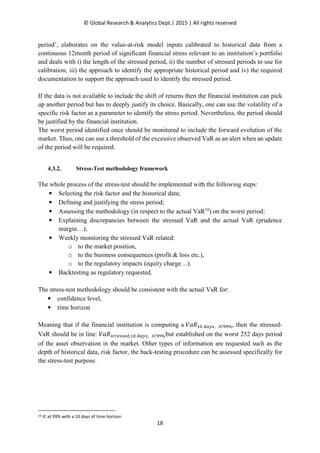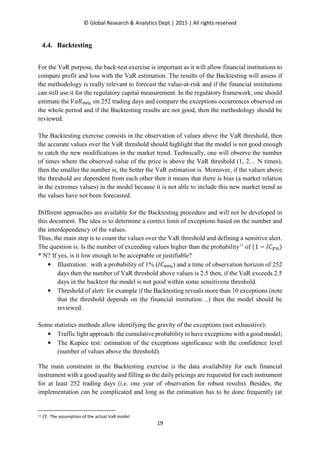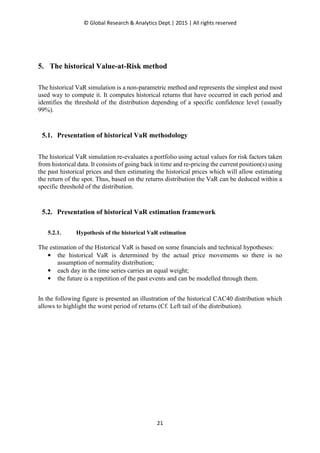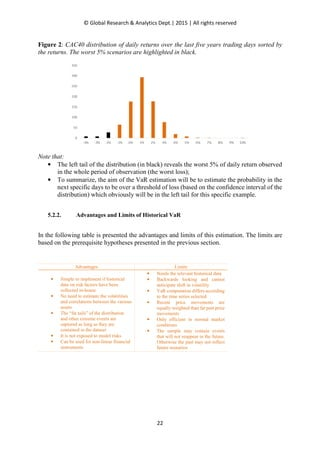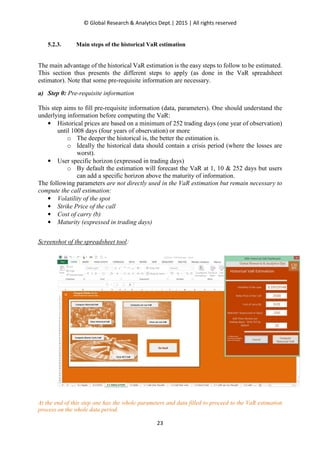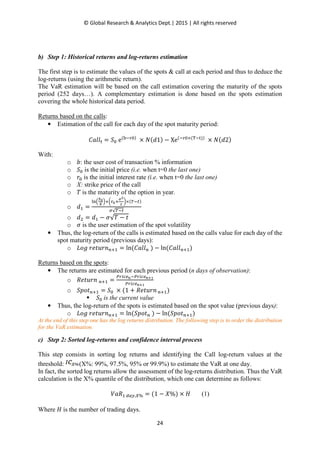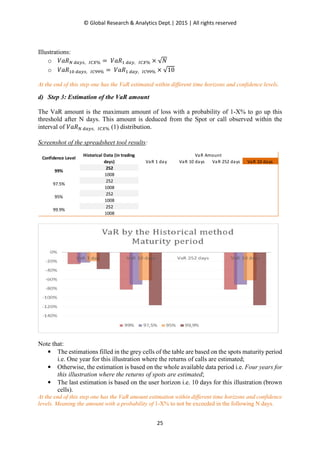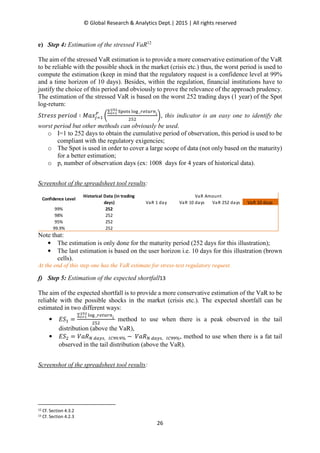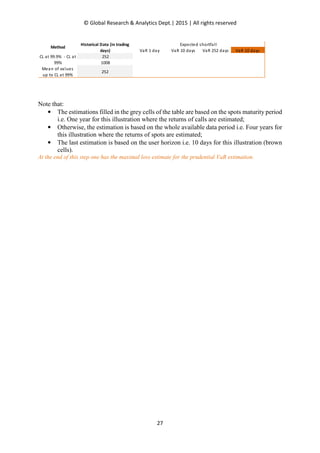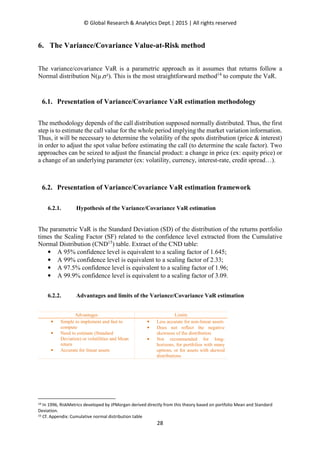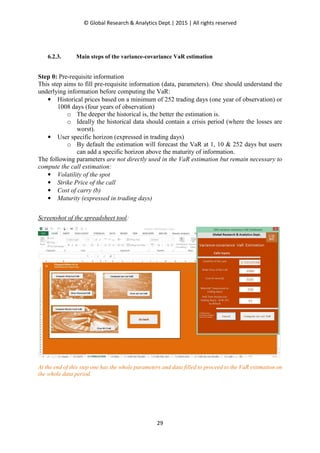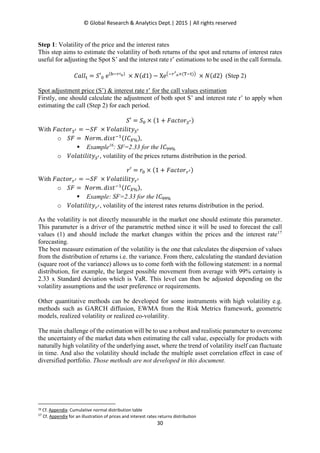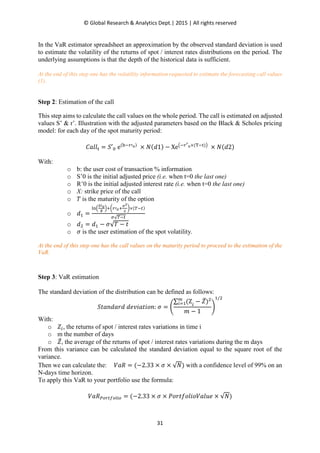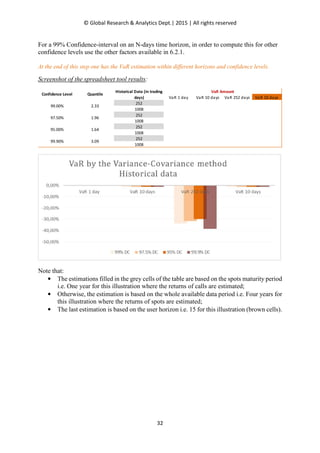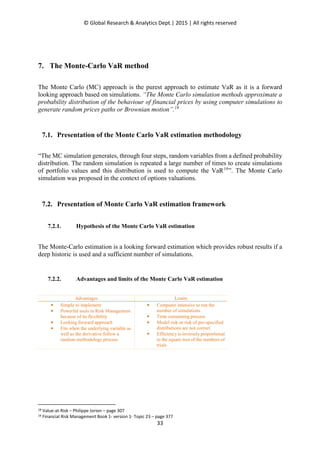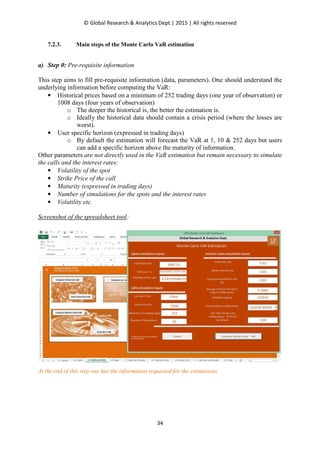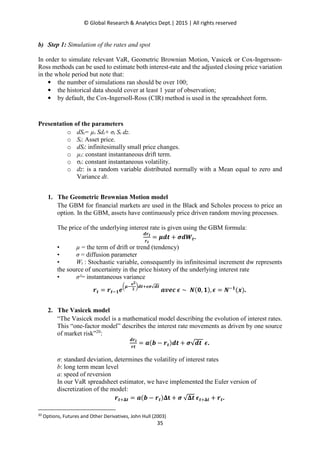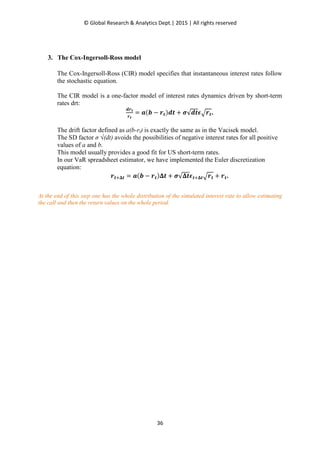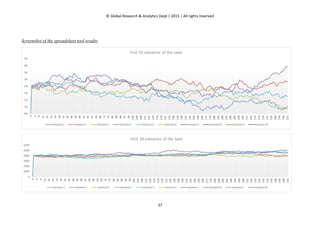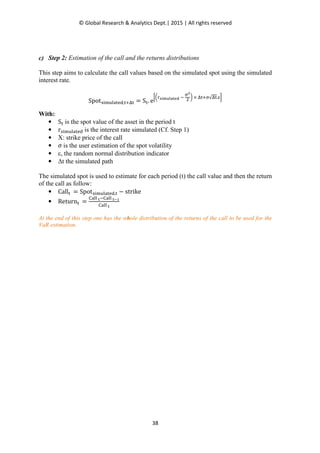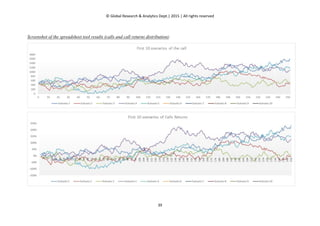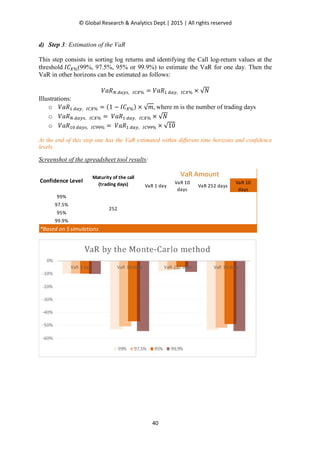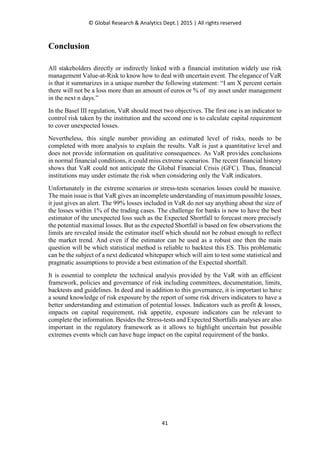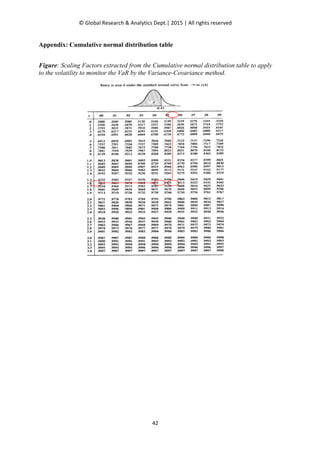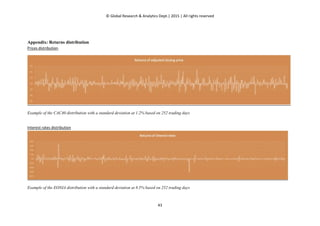Upcoming SlideShare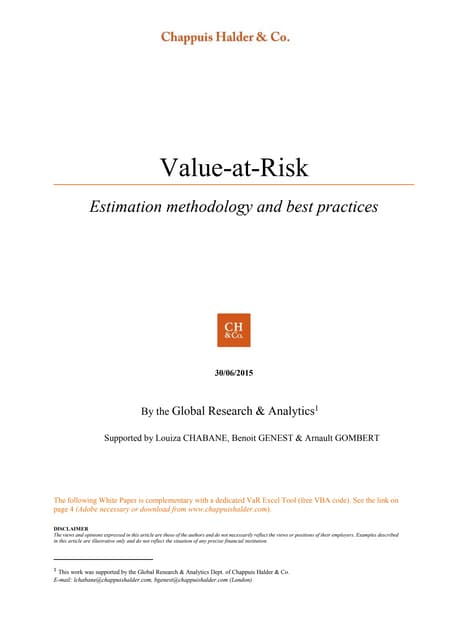CH&CO - VaR methodology whitepaper - 2015
1 of 44

### Chappuis Halder & Co - VaR estimation solutions

1. Value-at-Risk Estimation methodology and best practices 30/06/2015 By the Global Research & Analytics1 Supported by Louiza CHABANE, Benoit GENEST & Arnault GOMBERT The following White Paper is complementary with a dedicated VaR Excel Tool (free VBA code). See the link on page 4 (Adobe necessary or download from www.chappuishalder.com). DISCLAIMER The views and opinions expressed in this article are those of the authors and do not necessarily reflect the views or positions of their employers. Examples described in this article are illustrative only and do not reflect the situation of any precise financial institution. 1 This work was supported by the Global Research & Analytics Dept. of Chappuis Halder & Co. E-mail: lchabane@chappuishalder.com, bgenest@chappuishalder.com (London)
2. © Global Research & Analytics Dept.| 2015 | All rights reserved 2 Table of contents Vale at Risk : Estimation methodlogy and best practices ....................................................................... 4 1. Abstract ........................................................................................................................................... 4 2. What’s the Value-at-Risk (VaR)? ................................................................................................... 5 2.1. Short history of VaR and Capital Adequacy Requirement...................................................... 5 2.2. What’s the VaR about?............................................................................................................ 6 2.3. The purpose of VaR ................................................................................................................ 6 2.4. What’s the VaR for?................................................................................................................ 7 2.5. The required hypothesis to estimate the VaR.......................................................................... 7 3. Post-crisis VaR measure regulatory framework.............................................................................. 9 3.1. Current market risk framework ............................................................................................... 9 3.1.1. Basel 2.5.......................................................................................................................... 9 3.1.2. Stressed VaR.................................................................................................................. 9 3.1.3. IRC Incremental Risk Charge ................................................................................... 10 3.1.4. Comprehensive Risk Measure.................................................................................... 10 3.2. Fundamental Review of the Trading Book - FRTB .............................................................. 10 3.3. Basel 3................................................................................................................................... 10 4. Presentation of the VaR framework methodology ........................................................................ 12 4.1. The three main methodologies to compute VaR................................................................... 12 4.1.1. Presentation ................................................................................................................. 12 4.1.2. Basic indicators............................................................................................................ 12 4.1.3. Quantitative methods.................................................................................................. 13 4.1.4. Main common steps of a VaR estimation.................................................................. 14 4.2. Expected Shortfall................................................................................................................. 14 4.2.1. Expected shortfall motivations................................................................................... 14 4.2.2. Expected shortfall applications .................................................................................. 15 4.2.3. Expected shortfall practical approach....................................................................... 15 4.3. Stress-Test ............................................................................................................................. 17 4.3.1. Stress-Test regulatory framework............................................................................. 17 4.3.2. Stress-Test methodology framework ......................................................................... 18 4.4. Backtesting............................................................................................................................ 19 5. The historical Value-at-Risk method............................................................................................. 21 5.1. Presentation of historical VaR methodology......................................................................... 21 5.2. Presentation of historical VaR estimation framework........................................................... 21
3. © Global Research & Analytics Dept.| 2015 | All rights reserved 3 5.2.1. Hypothesis of the historical VaR estimation............................................................. 21 5.2.2. Advantages and Limits of Historical VaR................................................................. 22 5.2.3. Main steps of the historical VaR estimation ............................................................. 23 6. The Variance/Covariance Value-at-Risk method.......................................................................... 28 6.1. Presentation of Variance/Covariance VaR estimation methodology .................................... 28 6.2. Presentation of Variance/Covariance VaR estimation framework........................................ 28 6.2.1. Hypothesis of the Variance/Covariance VaR estimation......................................... 28 6.2.2. Advantages and limits of the Variance/Covariance VaR estimation...................... 28 6.2.3. Main steps of the variance-covariance VaR estimation........................................... 29 7. The Monte-Carlo VaR method...................................................................................................... 33 7.1. Presentation of the Monte Carlo VaR estimation methodology............................................ 33 7.2. Presentation of Monte Carlo VaR estimation framework ..................................................... 33 7.2.1. Hypothesis of the Monte Carlo VaR estimation....................................................... 33 7.2.2. Advantages and limits of the Monte Carlo VaR estimation .................................... 33 7.2.3. Main steps of the Monte Carlo VaR estimation........................................................ 34 Presentation of the parameters .......................................................................................................... 35 1. The Geometric Brownian Motion model .................................................................................. 35 2. The Vasicek model.................................................................................................................... 35 3. The Cox-Ingersoll-Ross model.................................................................................................. 36 Conclusion............................................................................................................................................. 41 Appendix: Cumulative normal distribution table.................................................................................. 42 Appendix: Returns distribution ............................................................................................................. 43
5. © Global Research & Analytics Dept.| 2015 | All rights reserved 5 2. What’s the Value-at-Risk (VaR)? 2.1. Short history of VaR and Capital Adequacy Requirement Following the 1980’s financial disasters and especially the 1987 October stock market crash and the increasing influence of mathematics and statistics modelling, the emergence of the dedicated quantitative methodologies revolutionised the financial risk industry. The VaR became, during the 90’s, the industry standard to express market risk indicator. In 1988, the Basel Accord, aka Basel I Accord, defines for the first time a minimum capital requirement, the Cook ratio, to cover losses incurred with Credit Risk. Banks must maintain risk capital at least 8% of their total Risk Weighted Assets (RWA). The “Cook ratio” or solvency ratio was created: ‫݋݅ݐܽݎ ݇݋݋ܥ‬ ൌ ஼௔௣௜௧௔௟ ஺ௗ௘௤௨௔௖௬ ோௐ஺಴ೝ೐೏೔೟ ೃ೔ೞೖ ൒ 8%. The Cook ratio was highly criticized as it only took into account Credit Risk for the capital adequacy computation. In 1996, the amended Basel I Accord includes Market Risks to monitor market exposure. Based on statistical techniques the VaR was, firstly, built to meet this requirement. The VaR was developed to monitor impacts of four parameters on financial product: interest- rate, commodity, equity price and currency. • Interest-rate risk: the risk that interest rates (i.e. Libor, Euribor, etc.) will change. • Commodity risk: the risk that commodity prices (i.e. corn, copper, crude oil, etc.) will change. • Equity risk: the risk that stock or stock indices (i.e. Euro Stoxx 50, etc.) prices will change. • Currency risk: the risk that foreign exchange rates (i.e. EUR/USD, EUR/GBP, etc.) will change. The Basel Committee agreed for financial institutions to use their internal validated VaR models. In return, banking regulation rules enforce banks to backtest them. In this new regulatory framework, the notion of VaR was mainly democratized by JP Morgan through its RiskMetrics software. The key contribution of the service was that it made the parametric or variance/covariance VaR tool freely available to anyone. In 2004, Basel Committee published a new Accord that enforces banks to stress their valuation models and enable capital requirement to cover Credit, Market and Operational risk. The more complete solvency McDonough ratio replaced the initial Cook ratio.
6. © Global Research & Analytics Dept.| 2015 | All rights reserved 6 ‫݋݅ݐܽݎ ݄݃ݑ݋݊݋ܦܿܯ‬ ൌ ஼௔௣௜௧௔௟ ஺ௗ௘௤௨௔௖௬ ோௐ஺಴ೝ೐೏೔೟ ೃ೔ೞೖାோௐ஺ಾೌೝೖ೐೟ ೃ೔ೞೖାோௐ஺ೀ೛೐ೌ೟೔೚೙ೌ೗ ೃ೔ೞೖ ൒ 8%. From that time, the VaR has been declined into a large range of quantitative risk indicators: Stress-tests, Back-testing, Expected Tail Losses… 2.2. What’s the VaR about? “The Value-at-Risk (VaR) defines 1 a probabilistic method of measuring the potential loss in portfolio value over a given time period and for a given distribution of historical returns. VaR is expressed in dollars or percentage losses of a portfolio (asset) value that will be equalled or exceeded only X percent of the time. In other words, there is an X percent probability that the loss in portfolio value will be equal to or greater than the VaR measure. For instance, assume a risk manager performing the daily 5% VaR as \$10,000. The VaR (5%) of \$10,000 indicates that there is a 5% of chance that on any given day, the portfolio will experience a loss of \$10,000 or more.” Most of the time, VaR is computed daily and estimated between today and tomorrow. Figure 1: Probability distribution of a Value-at-Risk with 95% Confidence Level and 1 day Time Horizon (Parametric VaR expressed as with a Normal Law N(0,1)). 2.3. The purpose of VaR The elegance of VaR is that it is easy to understand for internal and external stakeholders. Conventionally reported as a positive number VaR tends to mark a boundary between normal days and extreme events. 1 Financial Risk Management book 1, Foundations of Risk Management; Quantitative Analysis, page 23.
8. © Global Research & Analytics Dept.| 2015 | All rights reserved 8 Basically, to estimate the N days VaR from a 1 day result we consider that the N days VaR is equal to the square root of N multiplied by the VaR 1 day. ܸܴܽሺ99%, ܰ ݀ܽ‫ݏݕ‬ሻ ൌ ܸܴܽሺ99%, 1 ݀ܽ‫ݕ‬ሻ × √ܰ
9. © Global Research & Analytics Dept.| 2015 | All rights reserved 9 3. Post-crisis VaR measure regulatory framework The VaR estimation is extensively used by regulators and financial institutions to estimate regulatory capital or for internal monitoring. They usually use the 10-day 99% VaR for market risk and 1-year 99.9% for credit and operational risk. In the following sections, we’ll focus on the market VaR since it’s the main measure for market risk and as such has been subject to a number of regulatory initiatives in recent years. 3.1. Current market risk framework The current market risk framework is a resultant of successive regulations with purpose to address identified shortfalls of the in force. 3.1.1. Basel 2.5 In the aftermath of the 2008 financial crisis, changes were necessary to the calculation of capital for market risk in the Basel 2 framework with regard to the huge losses incurred by Financial Institutions relative to their trading book. Those changes were referred to as Basel 2.5, with their implementation realised in December 31th 2011: 3 additional measures were introduced in addition to the traditional VaR: • Stressed VaR calculation; • A new Incremental risk Charge (IRC); • A Comprehensive Risk Measure for credit correlation. 3.1.2. Stressed VaR In Basel 1, the VaR estimation was the 10-day at 99% VaR. Most banks using the historical VaR, based on the fact that the low volatilities in the 2003-2006 period were not able to anticipate sudden high level of VaRs after 2007. Therefore, to factor potential periods of stress, the Basel Committee introduced the stressed VaR in order to have a VaR estimated in a stressed period and a robust one. The historical simulation estimation assumes that the percentages of changes in market variables during the next days are a sample random from their percentages of daily changes observed during the 250-day period of stressed market conditions. The additional stressed VaR requirement should help reduce the pro-cyclicality of the minimum capital requirement for the market risk purpose.
11. © Global Research & Analytics Dept.| 2015 | All rights reserved 11 are computed. These can be used to add the counterparty’s CVA to the other positions considered for market-risk calculations.
12. © Global Research & Analytics Dept.| 2015 | All rights reserved 12 4. Presentation of the VaR framework methodology 4.1. The three main methodologies to compute VaR 4.1.1. Presentation In this document, a focus will be done on the three main methods used by financial institutions: • Historical estimation – Not a parametric method • Variance-Covariance estimation – Parametric: linear method • Monte-Carlo estimation – Not a parametric method Besides, before detailing the different methods, a short presentation of the basics relevant indicators implied in the VaR estimation is done to help the reader understand the technical terms. 4.1.2. Basic indicators The main indicator is the confidence level which is the probability scale for the loss to not exceed a specific VaR amount within a time horizon: • 99% (as the regulatory request for the estimation horizon); • 97.5%; • 95%; • 99.9% (as the regulatory request for the stress-test horizon). This scale is often associated to a time horizon (trading days) of forecasting period of the VaR: • 1 day; • 10 days (as the regulatory request for the estimation and stress-test horizon); • 252 days (one year); • The user time horizon. Note that to be confident with the results, a minimum of 252 trading days historic of observations (i.e. one year) is requested. The main benefit of VAR calculation is the diversification effects between financial instruments in the same portfolio. “Risk is reduced by investing in various assets. If the assets value do not move up and down in perfect synchrony, a diversified portfolio will be less risky than the weighted average risk of its constituent assets, and often less risk than the least risky of its constituents4 ”: • If securities are perfectly correlated (correlation of 1) the portfolio VaR of several securities equals the VaR for the individual positions. • If securities have no correlation (correlation of 0) the portfolio VaR is fully diversified and the risk equals to zero. • A correlation between 0 and 1 drives to a diversified risk estimated depending on the level of correlation between financial instruments. 4 Sullivan, Arthur; Steven M. Sheffrin (2003). Economics: principles in action. Upper saddle river, New Jersey 07458: Pearson Prentice Gall. P273
13. © Global Research & Analytics Dept.| 2015 | All rights reserved 13 4.1.3. Quantitative methods a) Linear method assumes the normality of the distributions of the returns Parametric VaR (Variance/ Covariance, Delta-valuation, correlation method): Parametric approach assumes the normality of the returns. Correlations between risk factors are constant and the delta (or price sensitivity to changes in a risk factor) of each portfolio constituent is constant. Using the correlation method, the Standard Deviation (volatility) of each risk factor is extracted from the historical observation period. The potential effect of each component of the portfolio on the overall portfolio value is then worked out from the component’s delta (with respect to a particular risk factor) and that risk factor’s volatility. b) Two Full-Valuation methods exist based on the initial position measure risk re-pricing the portfolio over a range of scenarios • Historical VaR: Historical VaR involves running the current portfolio across a set of historical price changes to yield a distribution of changes in portfolio value and computing a percentile. • Monte-Carlo VaR: Monte Carlo does not assume normality of the distribution. It is based on historical observations. A large number of randomly generated simulations are run forward in time using volatility and correlations estimations chosen by the risk manager. Each simulation will be different but in total the simulations will aggregate to the chosen statistical parameters. c) Other Conditional methodologies exist5: • Exponentially Weighted Moving Average (EWMA) model; • Autoregressive Conditional Heteroskedasticity (ARCH) models; • The declined (G)ARCH (1,1) model. d) Stress Test The reinforcement of the regulatory framework imposes a stress-test of the VaR and an estimation of the expected shortfall: • The stressed VaR should be estimated periodically based on one worst year of observation within ten days of horizon and a confidence level at 99.9% (i.e. above the 99% threshold for the actual VaR) • The expected shortfall will represent the maximal amount of losses not included in the VaR threshold (above the 99% threshold) and will allow financial institutions to have a deeper view of the risk. Note that these estimations are available in the VaR spreadsheet estimator and the methods overview will be developed in the following sections. e) VaR regulatory Backtesting exercise As for the Basel indicators, the VaR should be controlled periodically with a specific methodology to assess the performance and the relevance of the estimation. This exercise will not be developed in the VaR spreadsheet estimator nevertheless, a short presentation of the exercise is done in this document to better understand the main steps and what is at stake. 5 Not exhaustive and the methodologies are not detailed in this document
14. © Global Research & Analytics Dept.| 2015 | All rights reserved 14 4.1.4. Main common steps of a VaR estimation To compute a VaR, the methodology always follows the same process independently of the approach: • Mark to market the value of the portfolio at the initial time (last date); • Measure the variability of the risk factors; • Set a time horizon to estimate the VaR results (1 day, 10 days…); • Define a confidence level depending on the level of risk of the portfolio (95%, 99%...); • Report the “worst potential losses over a Time Horizon with a certain confidence level” using a probabilistic distribution of revenues. In the following sections are presented the Expected Shortfall, the stress-test and Backtesting approaches. The actual VaR estimations are developed in the sections: • 5] The historical Value-at-Risk method, • 6] The Variance/Covariance Value-at-Risk method, • 7] The Monte-Carlo VaR method. 4.2. Expected Shortfall The Expected Shortfall method estimates losses when an observed return is beyond the VaR. Above all, this measure is used when the returns distribution has fat tails or when the distribution beyond the VaR looks unusual. But also when one has a non-linear position for instance with options. “For example, for a probability level of 99%, a VaR equal to \$1 million means that the loss of the position should not exceed \$1 million in 99 cases out of 100 on average. A natural question is how much a position can lose on the exceptional hundredth case.”6 4.2.1. Expected shortfall motivations When one forecasts a VaR spreadsheet estimator, he estimates a value. This value represents the worst expected loss of a position for a determined time horizon. For example, when we take the case used in the variance/covariance part, we calculated a VaR at a 95% confidence level with a time horizon of one day. Unfortunately, these methods cannot measure, if we assume a return is exceeding the VaR, how far this exceeding return would be from the VaR. Statistically speaking, this is how we define the expected shortfall for a confidence level (α) with a specific time horizon (d): ‫ܵܧ‬ఈ,ௗ ൌ ॱሾ݈‫ݏݏ݋݈|ݏݏ݋‬ ≤ −ܸܴܽሺߙ, ݀ሻሿ. The main downside lies in the weakness of the estimation. Indeed, when one wants to implement the expected shortfall with a historical distribution, there are just a few observations 6 Source: Beyond the VaR – Longin 2001.
15. © Global Research & Analytics Dept.| 2015 | All rights reserved 15 beyond the VaR. Thus, one needs to be careful with the estimation that can be called a risky one, because of the lack of robustness of the estimator. However, nowadays, it is the best estimation we have in order to prevent losses from exceeding the VaR. 4.2.2. Expected shortfall applications 4.2.2.1. Returns distribution with fat tails The variance/covariance method assumes the returns distribution follows a normal one. So the 5% quantile of this normal distribution was taken into account in order to determine the VaR. Overall, when one exploits linear positions, the returns distribution could be computed. Thus, with enough historical data, one should be able to determine the distribution of the tails. The dispersion beyond the VaR is important, the fatter the tail is the biggest a loss could be when a return exceeds the VaR value. With the assumption of a normal distribution, the tail is thin, so the losses would be condensed around the VaR. 4.2.2.2. Positions with options In a matter of non-linear positions: leverages positions and highly leveraged positions may, when a return exceeds the VaR, bring about great losses. For instance, let’s say we take a short position on the underlying asset, we buy n put options and sell n call options. We bought put options thanks to the sale of the call options. Let’s call P the price of the position. ܲ ൌ ܵ଴ − ்ܵ − ݊ × maxሺ்ܵ − ‫ܭ‬௖௔௟௟, 0ሻ + ݊ × max൫‫ܭ‬௣௨௧ − ்ܵ, 0൯. Where S0 is the initial price of the underlying asset, let’s fix it to the amount of \$1000. We assume the returns follows a normal distribution. Thus, the 95% quantile is fixed to the amount of \$1016.45. Moreover, we assume the value of the put call is exactly the value of the normal distribution’s 95% quantile. So, the strike value of the call is equal to the VaR (95%). When we compare the loss beyond the VaR with a linear position, we can see that the dispersion above the VaR is more important than the linear position. 4.2.3. Expected shortfall practical approach As we said in the first paragraph, the expected shortfall could be a risky estimator because there would be few returns which would exceed the VaR. Thus, an approach to this problem was to be more conservative with the VaR. Instead of having a 99% VaR, Longin7 recommends to put 7 Source: Beyond the VaR – Longin 2001.
16. © Global Research & Analytics Dept.| 2015 | All rights reserved 16 a 99.9% VaR. This method would cover even more exceeding returns beyond the VaR. But, we come back to the first problem about measuring the loss beyond the VaR. Nevertheless, we reckon choosing between a 99.9% VaR and the expected shortfall depends on the tail distribution and the distance between observed returns beyond the VaR and the VaR. We think some tails imply the inevitable use of a unique method. 4.2.3.1. Unusual tails When a distribution is close to a normal one, the expected shortfall is a good approach because of the condensed distribution around the VaR. Nevertheless, one could imagine a distribution with a peak in the tail such as the chart beneath. In this case, the density distribution makes us think if a return is beyond the VaR, this return has more chances to be near the mathematical expectation of the peak. If we compute the expected shortfall, the value estimated could be less than this mathematical expectation which means one may underestimate the loss. This example highlights the limit of the expected shortfall method. Indeed, in some cases, it would clearly underestimate the loss of a return. In order to overcome this limit, we propose to adopt a threshold approach to determine when the expected shortfall should be used. 4.2.3.2. A threshold approach We pointed out the fact the expected shortfall could underestimate the loss. In this case, we advise to use a more conservative approach, which means using a 99.9% VaR. Nevertheless, when we concentrate on the distribution tail beyond the VaR the expected shortfall must be measured in order to choose between a more conservative VaR and the expected shortfall method. The choice is based on thresholds set up by the bank’s cover strategy. We have to take into account the tail of the returns distribution where one would face two scenarios: • A peak in the tail distribution, • Or a fat tail. 4.2.3.3. A peak in the tail If the tail distribution, after a 99% estimated VaR, includes a “peak”, one may calculate the difference between the mean of the peak in the tail and the expected shortfall, as the expected shortfall must underestimate the loss which is concentrated around the mean of the peak. A different threshold must be computed by the bank in order to choose between a more conservative VaR, if the difference is not so far in terms of loss, or to keep the same VaR, because a more conservative VaR would not cover the peak and in a matter of capital
17. © Global Research & Analytics Dept.| 2015 | All rights reserved 17 requirements a 99% quantile of the peak distribution would be extremely costly. The formula can be written as follow: ‫ܵܧ‬ଵ ൌ 1 ∑ ૤൛௟௢௚௥௘௧௨௥௡೔ழ௏௔ோಿ ೏ೌ೤ೞ,಺಴వవ%ൟ ଶହଶ ௜ୀଵ ෍ ݈‫݊ݎݑݐ݁ݎ݃݋‬௜૤൛௟௢௚௥௘௧௨௥௡೔ழ௏௔ோಿ ೏ೌ೤ೞ,಺಴వవ%ൟ ଶହଶ ௜ୀଵ 4.2.3.4. A fat tail (without a peak) If one considers the tail distribution as fat, meaning fatter than the tail of a normal distribution, one may compute the expected shortfall and may measure the difference between the expected shortfall and the VaR. One may compute an indicator which would be defined as a ratio, the numerator would be the difference, and the estimated VaR as the denominator. The greater the ratio is, the more concentrated around the expected shortfall the losses are. Thus, a bank would need to define a threshold on the ratio in order to determine if the VaR spreadsheet estimator is conservative enough or if they have to use a more conservative VaR. The expected shortfall is more coherent than the actual VaR estimation nevertheless, the implementation and the Backtesting of this indicator is not as simple as for the actual VaR. Besides, it is more of a concern for credit risk and operational risk than for market risk (in general market distribution can be approximated with a normal distribution). The formula can be written as follows: ‫ܵܧ‬ଶ = ܸܴܽே ௗ௔௬௦, ூ஼ଽଽ.ଽ% − ܸܴܽே ௗ௔௬௦, ூ஼ଽଽ% 4.3. Stress-Test 4.3.1. Stress-Test regulatory framework The VaR stress-test is summited to regulatory exigencies for the internal model to be Basel compliant. Thus, some guidelines are suggested by the European regulatory to be controlled by the local regulators. The main exigency for the VaR model is to select a cumulative period of at least 12 months which includes the worst period8 : “The requirement set out in the CRD that the historical data used to calibrate the Stressed VaR measure have to cover a continuous 12- month period, applies also where institutions identify a period which is shorter than 12 months but which is considered to be a significant stress event relevant to an institution’s portfolio”. Note that the scenario for the non-VaR models9 are not presented in this document. No mandatory process is suggested for the worst period identification but the identification of the period should be detailed and justified, extract of the EBA Guidelines on Stressed Value At Risk (Stressed VaR) EBA/GL/2012/2: … on ‘Identification and validation of the stressed 8 Cf. EBA guidelines on Stressed Value At Risk – EBA /GL / 2012 / 2 9 Cf. www.eba.com
18. © Global Research & Analytics Dept.| 2015 | All rights reserved 18 period’, elaborates on the value-at-risk model inputs calibrated to historical data from a continuous 12month period of significant financial stress relevant to an institution’s portfolio and deals with i) the length of the stressed period, ii) the number of stressed periods to use for calibration, iii) the approach to identify the appropriate historical period and iv) the required documentation to support the approach used to identify the stressed period. If the data is not available to include the shift of returns then the financial institution can pick up another period but has to deeply justify its choice. Basically, one can use the volatility of a specific risk factor as a parameter to identify the stress period. Nevertheless, the period should be justified by the financial institution. The worst period identified once should be monitored to include the forward evolution of the market. Thus, one can use a threshold of the excessive observed VaR as an alert when an update of the period will be required. 4.3.2. Stress-Test methodology framework The whole process of the stress-test should be implemented with the following steps: • Selecting the risk factor and the historical data; • Defining and justifying the stress period; • Assessing the methodology (in respect to the actual VaR10 ) on the worst period; • Explaining discrepancies between the stressed VaR and the actual VaR (prudence margin…); • Weekly monitoring the stressed VaR related: o to the market position, o to the business consequences (profit & loss etc.), o to the regulatory impacts (equity charge…). • Backtesting as regulatory requested. The stress-test methodology should be consistent with the actual VaR for: • confidence level, • time horizon Meaning that if the financial institution is computing a ܸܴܽଵ଴ ௗ௔௬௦, ூ஼ଽଽ%, then the stressed- VaR should be in line: ܸܴܽ௦௧௥௘௦௦௘ௗ,ଵ଴ ௗ௔௬௦, ூ஼ଽଽ%but established on the worst 252 days period of the asset observation in the market. Other types of information are requested such as the depth of historical data, risk factor, the back-testing procedure can be assessed specifically for the stress-test purpose. 10 IC at 99% with a 10 days of time horizon
19. © Global Research & Analytics Dept.| 2015 | All rights reserved 19 4.4. Backtesting For the VaR purpose, the back-test exercise is important as it will allow financial institutions to compare profit and loss with the VaR estimation. The results of the Backtesting will assess if the methodology is really relevant to forecast the value-at-risk and if the financial institutions can still use it for the regulatory capital measurement. In the regulatory framework, one should estimate the ܸܴܽଽଽ% on 252 trading days and compare the exceptions occurrences observed on the whole period and if the Backtesting results are not good, then the methodology should be reviewed. The Backtesting exercise consists in the observation of values above the VaR threshold, then the accurate values over the VaR threshold should highlight that the model is not good enough to catch the new modifications in the market trend. Technically, one will observe the number of times where the observed value of the price is above the VaR threshold (1, 2… N times), then the smaller the number is, the better the VaR estimation is. Moreover, if the values above the threshold are dependent from each other then it means that there is bias (a market relation in the extremes values) in the model because it is not able to include this new market trend as the values have not been forecasted. Different approaches are available for the Backtesting procedure and will not be developed in this document. The idea is to determine a correct limit of exceptions based on the number and the interdependency of the values. Thus, the main step is to count the values over the VaR threshold and defining a sensitive alert. The question is: Is the number of exceeding values higher than the probability11 of (1 − ‫ܥܫ‬௑%) * N? If yes, is it low enough to be acceptable or justifiable? • Illustration: with a probability of 1% (‫ܥܫ‬ଽଽ%) and a time of observation horizon of 252 days then the number of VaR threshold above values is 2.5 then, if the VaR exceeds 2.5 days in the backtest the model is not good within some sensitivene threshold. • Threshold of alert: for example if the Backtesting reveals more than 10 exceptions (note that the threshold depends on the financial institution…) then the model should be reviewed. Some statistics methods allow identifying the gravity of the exceptions (not exhaustive): • Traffic light approach: the cumulative probability to have exceptions with a good model; • The Kupiec test: estimation of the exceptions significance with the confidence level (number of values above the threshold). The main constraint in the Backtesting exercise is the data availability for each financial instrument with a good quality and filling as the daily pricings are requested for each instrument for at least 252 trading days (i.e. one year of observation for robust results). Besides, the implementation can be complicated and long as the estimation has to be done frequently (at 11 Cf. The assumption of the actual VaR model
20. © Global Research & Analytics Dept.| 2015 | All rights reserved 20 least, once per week). Nevertheless, this exercise is vital if one wants to attend to an internal model regulatory process.
21. © Global Research & Analytics Dept.| 2015 | All rights reserved 21 5. The historical Value-at-Risk method The historical VaR simulation is a non-parametric method and represents the simplest and most used way to compute it. It computes historical returns that have occurred in each period and identifies the threshold of the distribution depending of a specific confidence level (usually 99%). 5.1. Presentation of historical VaR methodology The historical VaR simulation re-evaluates a portfolio using actual values for risk factors taken from historical data. It consists of going back in time and re-pricing the current position(s) using the past historical prices and then estimating the historical prices which will allow estimating the return of the spot. Thus, based on the returns distribution the VaR can be deduced within a specific threshold of the distribution. 5.2. Presentation of historical VaR estimation framework 5.2.1. Hypothesis of the historical VaR estimation The estimation of the Historical VaR is based on some financials and technical hypotheses: • the historical VaR is determined by the actual price movements so there is no assumption of normality distribution; • each day in the time series carries an equal weight; • the future is a repetition of the past events and can be modelled through them. In the following figure is presented an illustration of the historical CAC40 distribution which allows to highlight the worst period of returns (Cf. Left tail of the distribution).
22. © Global Research & Analytics Dept.| 2015 | All rights reserved 22 Figure 2: CAC40 distribution of daily returns over the last five years trading days sorted by the returns. The worst 5% scenarios are highlighted in black. Note that: • The left tail of the distribution (in black) reveals the worst 5% of daily return observed in the whole period of observation (the worst loss); • To summarize, the aim of the VaR estimation will be to estimate the probability in the next specific days to be over a threshold of loss (based on the confidence interval of the distribution) which obviously will be in the left tail for this specific example. 5.2.2. Advantages and Limits of Historical VaR In the following table is presented the advantages and limits of this estimation. The limits are based on the prerequisite hypotheses presented in the previous section. Advantages Limits • Simple to implement if historical data on risk factors have been collected in-house • No need to estimate the volatilities and correlations between the various assets • The “fat tails” of the distribution and other extreme events are captured as long as they are contained in the dataset • It is not exposed to model risks • Can be used for non-linear financial instruments • Needs the relevant historical data • Backwards looking and cannot anticipate shift in volatility • VaR computation differs according to the time series selected • Recent price movements are equally weighted than far past price movements • Only efficient in normal market conditions • The sample may contain events that will not reappear in the future. Otherwise the past may not reflect future scenarios
24. © Global Research & Analytics Dept.| 2015 | All rights reserved 24 b) Step 1: Historical returns and log-returns estimation The first step is to estimate the values of the spots & call at each period and thus to deduce the log-returns (using the arithmetic return). The VaR estimation will be based on the call estimation covering the maturity of the spots period (252 days…). A complementary estimation is done based on the spots estimation covering the whole historical data period. Returns based on the calls: • Estimation of the call for each day of the spot maturity period: ‫݈݈ܽܥ‬୲ = ܵ଴ e(ୠି୰଴) × ܰ(݀1) − Xe(ି୰଴×(୘ି୲)) × ܰ(݀2) With: o ܾ: the user cost of transaction % information o ܵ଴ is the initial price (i.e. when t=0 the last one) o ‫ݎ‬଴ is the initial interest rate (i.e. when t=0 the last one) o X: strike price of the call o ܶ is the maturity of the option in year. o ݀ଵ = ୪୬ቀ ೄబ ೉ ቁା൬௥బା ഑మ మ ൰×(்ି௧) ఙ√்ି௧ o ݀ଶ = ݀ଵ − ߪ√ܶ − ‫ݐ‬ o ߪ is the user estimation of the spot volatility • Thus, the log-return of the calls is estimated based on the calls value for each day of the spot maturity period (previous days): o ‫݃݋ܮ‬ ‫݊ݎݑݐ݁ݎ‬௡ାଵ = ln(‫݈݈ܽܥ‬௡ ) − ln(‫݈݈ܽܥ‬௡ାଵ) Returns based on the spots: • The returns are estimated for each previous period (n days of observation): o ܴ݁‫݊ݎݑݐ‬ ௡ାଵ = ௉௥௜௖௘೙ି௉௥௜௖௘೙శభ ௉௥௜௖௘೙శభ o ܵ‫ݐ݋݌‬௡ାଵ = ܵ଴ × (1 + ܴ݁‫݊ݎݑݐ‬ ௡ାଵ) ܵ଴ is the current value • Thus, the log-return of the spots is estimated based on the spot value (previous days): o ‫݃݋ܮ‬ ‫݊ݎݑݐ݁ݎ‬௡ାଵ = ln(ܵ‫ݐ݋݌‬௡ ) − ln(ܵ‫ݐ݋݌‬௡ାଵ) At the end of this step one has the log returns distribution. The following step is to order the distribution for the VaR estimation. c) Step 2: Sorted log-returns and confidence interval process This step consists in sorting log returns and identifying the Call log-return values at the threshold: (X%: 99%, 97.5%, 95% or 99.9%) to estimate the VaR at one day. In fact, the sorted log returns allow the assessment of the log-returns distribution. Thus the VaR calculation is the X% quantile of the distribution, which one can determine as follows: ܸܴܽଵ ௗ௔௬,௑% = (1 − ܺ%) × ‫ܪ‬ (1) Where ‫ܪ‬ is the number of trading days.
25. © Global Research & Analytics Dept.| 2015 | All rights reserved 25 Illustrations: o ܸܴܽே ௗ௔௬௦, ூ஼௑% = ܸܴܽଵ ௗ௔௬, ூ஼௑% × √ܰ o ܸܴܽଵ଴ ௗ௔௬௦, ூ஼ଽଽ% = ܸܴܽଵ ௗ௔௬, ூ஼ଽଽ% × √10 At the end of this step one has the VaR estimated within different time horizons and confidence levels. d) Step 3: Estimation of the VaR amount The VaR amount is the maximum amount of loss with a probability of 1-X% to go up this threshold after N days. This amount is deduced from the Spot or call observed within the interval of ܸܴܽே ௗ௔௬௦, ூ஼௑% (1) distribution. Screenshot of the spreadsheet tool results: Note that: • The estimations filled in the grey cells of the table are based on the spots maturity period i.e. One year for this illustration where the returns of calls are estimated; • Otherwise, the estimation is based on the whole available data period i.e. Four years for this illustration where the returns of spots are estimated; • The last estimation is based on the user horizon i.e. 10 days for this illustration (brown cells). At the end of this step one has the VaR amount estimation within different time horizons and confidence levels. Meaning the amount with a probability of 1-X% to not be exceeded in the following N days. VaR 1 day VaR 10 days VaR 252 days VaR 10 days 252 1008 252 1008 252 1008 252 1008 95% 99.9% Confidence Level Historical Data (in trading days) VaR Amount 99% 97.5%
26. © Global Research & Analytics Dept.| 2015 | All rights reserved 26 e) Step 4: Estimation of the stressed VaR12 The aim of the stressed VaR estimation is to provide a more conservative estimation of the VaR to be reliable with the possible shock in the market (crisis etc.) thus, the worst period is used to compute the estimation (keep in mind that the regulatory request is a confidence level at 99% and a time horizon of 10 days). Besides, within the regulation, financial institutions have to justify the choice of this period and obviously to prove the relevance of the approach prudency. The estimation of the stressed VaR is based on the worst 252 trading days (1 year) of the Spot log-return: ܵ‫ݏݏ݁ݎݐ‬ ‫݀݋݅ݎ݁݌‬ ∶ ‫ݔܽܯ‬௝ୀଵ ௉ ൬ ∑ ୗ୮୭୲ୱ ୪୭୥ _௥௘௧௨௥௡మఱమ ೔సభ ೔ ଶହଶ ൰, this indicator is an easy one to identify the worst period but other methods can obviously be used. o I=1 to 252 days to obtain the cumulative period of observation, this period is used to be compliant with the regulatory exigencies; o The Spot is used in order to cover a large scope of data (not only based on the maturity) for a better estimation; o p, number of observation days (ex: 1008 days for 4 years of historical data). Screenshot of the spreadsheet tool results: Note that: • The estimation is only done for the maturity period (252 days for this illustration); • The last estimation is based on the user horizon i.e. 10 days for this illustration (brown cells). At the end of this step one has the VaR estimate for stress-test regulatory request. f) Step 5: Estimation of the expected shortfall13 The aim of the expected shortfall is to provide a more conservative estimation of the VaR to be reliable with the possible shocks in the market (crisis etc.). The expected shortfall can be estimated in two different ways: • ‫ܵܧ‬ଵ = ∑ ୪୭୥ _௥௘௧௨௥௡మఱమ ೔సభ ೔ ଶହଶ method to use when there is a peak observed in the tail distribution (above the VaR), • ‫ܵܧ‬ଶ = ܸܴܽே ௗ௔௬௦, ூ஼ଽଽ.ଽ% − ܸܴܽே ௗ௔௬௦, ூ஼ଽଽ%, method to use when there is a fat tail observed in the tail distribution (above the VaR). Screenshot of the spreadsheet tool results: 12 Cf. Section 4.3.2 13 Cf. Section 4.2.3 VaR 1 day VaR 10 days VaR 252 days VaR 10 days 99% 252 98% 252 95% 252 99.9% 252 Confidence Level Historical Data (in trading days) VaR Amount
27. © Global Research & Analytics Dept.| 2015 | All rights reserved 27 Note that: • The estimations filled in the grey cells of the table are based on the spots maturity period i.e. One year for this illustration where the returns of calls are estimated; • Otherwise, the estimation is based on the whole available data period i.e. Four years for this illustration where the returns of spots are estimated; • The last estimation is based on the user horizon i.e. 10 days for this illustration (brown cells). At the end of this step one has the maximal loss estimate for the prudential VaR estimation. VaR 1 day VaR 10 days VaR 252 days VaR 10 days 252 1008 Mean of values up to CL at 99% 252 Method Historical Data (in trading days) Expected shortfall CL at 99.9% - CL at 99%
28. © Global Research & Analytics Dept.| 2015 | All rights reserved 28 6. The Variance/Covariance Value-at-Risk method The variance/covariance VaR is a parametric approach as it assumes that returns follow a Normal distribution N(µ,σ²). This is the most straightforward method14 to compute the VaR. 6.1. Presentation of Variance/Covariance VaR estimation methodology The methodology depends of the call distribution supposed normally distributed. Thus, the first step is to estimate the call value for the whole period implying the market variation information. Thus, it will be necessary to determine the volatility of the spots distribution (price & interest) in order to adjust the spot value before estimating the call (to determine the scale factor). Two approaches can be seized to adjust the financial product: a change in price (ex: equity price) or a change of an underlying parameter (ex: volatility, currency, interest-rate, credit spread…). 6.2. Presentation of Variance/Covariance VaR estimation framework 6.2.1. Hypothesis of the Variance/Covariance VaR estimation The parametric VaR is the Standard Deviation (SD) of the distribution of the returns portfolio times the Scaling Factor (SF) related to the confidence level extracted from the Cumulative Normal Distribution (CND15 ) table. Extract of the CND table: • A 95% confidence level is equivalent to a scaling factor of 1.645; • A 99% confidence level is equivalent to a scaling factor of 2.33; • A 97.5% confidence level is equivalent to a scaling factor of 1.96; • A 99.9% confidence level is equivalent to a scaling factor of 3.09. 6.2.2. Advantages and limits of the Variance/Covariance VaR estimation Advantages Limits • Simple to implement and fast to compute • Need to estimate (Standard Deviation) or volatilities and Mean return • Accurate for linear assets • Less accurate for non-linear assets • Does not reflect the negative skewness of the distribution • Not recommended for long- horizons, for portfolios with many options, or for assets with skewed distributions 14 In 1996, RiskMetrics developed by JPMorgan derived directly from this theory based on portfolio Mean and Standard Deviation. 15 Cf. Appendix: Cumulative normal distribution table
29. © Global Research & Analytics Dept.| 2015 | All rights reserved 29 6.2.3. Main steps of the variance-covariance VaR estimation Step 0: Pre-requisite information This step aims to fill pre-requisite information (data, parameters). One should understand the underlying information before computing the VaR: • Historical prices based on a minimum of 252 trading days (one year of observation) or 1008 days (four years of observation) o The deeper the historical is, the better the estimation is. o Ideally the historical data should contain a crisis period (where the losses are worst). • User specific horizon (expressed in trading days) o By default the estimation will forecast the VaR at 1, 10 & 252 days but users can add a specific horizon above the maturity of information. The following parameters are not directly used in the VaR estimation but remain necessary to compute the call estimation: • Volatility of the spot • Strike Price of the call • Cost of carry (b) • Maturity (expressed in trading days) Screenshot of the spreadsheet tool: At the end of this step one has the whole parameters and data filled to proceed to the VaR estimation on the whole data period.
30. © Global Research & Analytics Dept.| 2015 | All rights reserved 30 Step 1: Volatility of the price and the interest rates This step aims to estimate the volatility of both returns of the spot and returns of interest rates useful for adjusting the Spot S’ and the interest rate r’ estimations to be used in the call formula. ‫݈݈ܽܥ‬୲ = ܵ′଴ e(ୠି௥ᇱబ) × ܰ(݀1) − Xe൫ି௥ᇲ బ×(୘ି୲)൯ × ܰ(݀2) (Step 2) Spot adjustment price (S’) & interest rate r’ for the call values estimation Firstly, one should calculate the adjustment of both spot S’ and interest rate r’ to apply when estimating the call (Step 2) for each period. ܵ′ = ܵ଴ × (1 + ‫ݎ݋ݐܿܽܨ‬ௌᇲ) With ‫ݎ݋ݐܿܽܨ‬ௌᇲ = −ܵ‫ܨ‬ × ܸ‫ݕݐ݈݅݅ݐ݈ܽ݋‬ௌᇲ o ܵ‫ܨ‬ = ܰ‫.݉ݎ݋‬ ݀݅‫ݐݏ‬ିଵ(‫ܥܫ‬௑%), Example16 : SF=2.33 for the ‫ܥܫ‬ଽଽ% o ܸ‫ݕݐ݈݅݅ݐ݈ܽ݋‬ௌᇲ, volatility of the prices returns distribution in the period. ‫′ݎ‬ = ‫ݎ‬଴ × (1 + ‫ݎ݋ݐܿܽܨ‬௥ᇲ) With ‫ݎ݋ݐܿܽܨ‬௥ᇲ = −ܵ‫ܨ‬ × ܸ‫ݕݐ݈݅݅ݐ݈ܽ݋‬௥ᇲ o ܵ‫ܨ‬ = ܰ‫.݉ݎ݋‬ ݀݅‫ݐݏ‬ିଵ(‫ܥܫ‬௑%), Example: SF=2.33 for the ‫ܥܫ‬ଽଽ% o ܸ‫ݕݐ݈݅݅ݐ݈ܽ݋‬௥ᇲ, volatility of the interest rates returns distribution in the period. As the volatility is not directly measurable in the market one should estimate this parameter. This parameter is a driver of the parametric method since it will be used to forecast the call values (1) and should include the market changes within the prices and the interest rate17 forecasting. The best measure estimation of the volatility is the one that calculates the dispersion of values from the distribution of returns i.e. the variance. From there, calculating the standard deviation (square root of the variance) allows us to come forth with the following statement: in a normal distribution, for example, the largest possible movement from average with 99% certainty is 2.33 x Standard deviation which is VaR. This level can then be adjusted depending on the volatility assumptions and the user preference or requirements. Other quantitative methods can be developed for some instruments with high volatility e.g. methods such as GARCH diffusion, EWMA from the Risk Metrics framework, geometric models, realized volatility or realized co-volatility. The main challenge of the estimation will be to use a robust and realistic parameter to overcome the uncertainty of the market data when estimating the call value, especially for products with naturally high volatility of the underlying asset, where the trend of volatility itself can fluctuate in time. And also the volatility should include the multiple asset correlation effect in case of diversified portfolio. Those methods are not developed in this document. 16 Cf. Appendix: Cumulative normal distribution table 17 Cf. Appendix for an illustration of prices and interest rates returns distribution
31. © Global Research & Analytics Dept.| 2015 | All rights reserved 31 In the VaR estimator spreadsheet an approximation by the observed standard deviation is used to estimate the volatility of the returns of spot / interest rates distributions on the period. The underlying assumptions is that the depth of the historical data is sufficient. At the end of this step one has the volatility information requested to estimate the forecasting call values (1). Step 2: Estimation of the call This step aims to calculate the call values on the whole period. The call is estimated on adjusted values S’ & r’. Illustration with the adjusted parameters based on the Black & Scholes pricing model: for each day of the spot maturity period: ‫݈݈ܽܥ‬୲ = ܵ′଴ e(ୠି௥ᇱబ) × ܰ(݀1) − Xe൫ି௥ᇲ బ×(୘ି୲)൯ × ܰ(݀2) With: o b: the user cost of transaction % information o S’0 is the initial adjusted price (i.e. when t=0 the last one) o R’0 is the initial adjusted interest rate (i.e. when t=0 the last one) o X: strike price of the call o ܶ is the maturity of the option o ݀ଵ = ୪୬ቀ ೄᇲబ ೉ ቁା൬௥ᇱబା ഑మ మ ൰×(்ି௧) ఙ√்ି௧ o ݀ଶ = ݀ଵ − ߪ√ܶ − ‫ݐ‬ o ߪ is the user estimation of the spot volatility. At the end of this step one has the call values on the maturity period to proceed to the estimation of the VaR. Step 3: VaR estimation The standard deviation of the distribution can be defined as follows: ܵ‫݀ݎܽ݀݊ܽݐ‬ ݀݁‫:݊݋݅ݐܽ݅ݒ‬ ߪ = ቆ ∑ (Z௠ ௜ୀଵ ௜ − ܼ̅)ଶ ݉ − 1 ቇ ଵ/ଶ With: o ܼ௜, the returns of spot / interest rates variations in time i o m the number of days o ܼ̅, the average of the returns of spot / interest rates variations during the m days From this variance can be calculated the standard deviation equal to the square root of the variance. Then we can calculate the: ܸܴܽ = (−2.33 × ߪ × √ܰ) with a confidence level of 99% on an N-days time horizon. To apply this VaR to your portfolio use the formula: ܸܴܽ௉௢௥௧௙௢௟௜௢ = (−2.33 × ߪ × ܲ‫݁ݑ݈ܸܽ݋݈݅݋݂ݐݎ݋‬ × √ܰ)
32. © Global Research & Analytics Dept.| 2015 | All rights reserved 32 For a 99% Confidence-interval on an N-days time horizon, in order to compute this for other confidence levels use the other factors available in 6.2.1. At the end of this step one has the VaR estimation within different horizons and confidence levels. Screenshot of the spreadsheet tool results: Note that: • The estimations filled in the grey cells of the table are based on the spots maturity period i.e. One year for this illustration where the returns of calls are estimated; • Otherwise, the estimation is based on the whole available data period i.e. Four years for this illustration where the returns of spots are estimated; • The last estimation is based on the user horizon i.e. 15 for this illustration (brown cells). VaR 1 day VaR 10 days VaR 252 days VaR 10 days 252 1008 252 1008 252 1008 252 1008 99.90% 3.09 Confidence Level Quantile Historical Data (in trading days) 99.00% 2.33 97.50% 1.96 95.00% 1.64 VaR Amount
33. © Global Research & Analytics Dept.| 2015 | All rights reserved 33 7. The Monte-Carlo VaR method The Monte Carlo (MC) approach is the purest approach to estimate VaR as it is a forward looking approach based on simulations. “The Monte Carlo simulation methods approximate a probability distribution of the behaviour of financial prices by using computer simulations to generate random prices paths or Brownian motion”.18 7.1. Presentation of the Monte Carlo VaR estimation methodology “The MC simulation generates, through four steps, random variables from a defined probability distribution. The random simulation is repeated a large number of times to create simulations of portfolio values and this distribution is used to compute the VaR19 ”. The Monte Carlo simulation was proposed in the context of options valuations. 7.2. Presentation of Monte Carlo VaR estimation framework 7.2.1. Hypothesis of the Monte Carlo VaR estimation The Monte-Carlo estimation is a looking forward estimation which provides robust results if a deep historic is used and a sufficient number of simulations. 7.2.2. Advantages and limits of the Monte Carlo VaR estimation Advantages Limits • Simple to implement • Powerful tools in Risk Management because of its flexibility • Looking forward approach • Fits when the underlying variable as well as the derivative follow a random methodology process • Computer intensive to run the number of simulations • Time consuming process • Model risk or risk of pre-specified distributions are not correct • Efficiency is inversely proportional to the square root of the numbers of trials 18 Value-at-Risk – Philippe Jorion – page 307 19 Financial Risk Management Book 1- version 1- Topic 23 – page 377
34. © Global Research & Analytics Dept.| 2015 | All rights reserved 34 7.2.3. Main steps of the Monte Carlo VaR estimation a) Step 0: Pre-requisite information This step aims to fill pre-requisite information (data, parameters). One should understand the underlying information before computing the VaR: • Historical prices based on a minimum of 252 trading days (one year of observation) or 1008 days (four years of observation) o The deeper the historical is, the better the estimation is. o Ideally the historical data should contain a crisis period (where the losses are worst). • User specific horizon (expressed in trading days) o By default the estimation will forecast the VaR at 1, 10 & 252 days but users can add a specific horizon above the maturity of information. Other parameters are not directly used in the VaR estimation but remain necessary to simulate the calls and the interest rates: • Volatility of the spot • Strike Price of the call • Maturity (expressed in trading days) • Number of simulations for the spots and the interest rates • Volatility etc. Screenshot of the spreadsheet tool: At the end of this step one has the information requested for the estimations.
35. © Global Research & Analytics Dept.| 2015 | All rights reserved 35 b) Step 1: Simulation of the rates and spot In order to simulate relevant VaR, Geometric Brownian Motion, Vasicek or Cox-Ingersson- Ross methods can be used to estimate both interest-rate and the adjusted closing price variation in the whole period but note that: • the number of simulations ran should be over 100; • the historical data should cover at least 1 year of observation; • by default, the Cox-Ingersoll-Ross (CIR) method is used in the spreadsheet form. Presentation of the parameters o dSt= µt Sdt+ σt St dz. o St: Asset price. o dSt: infinitesimally small price changes. o µt: constant instantaneous drift term. o σt: constant instantaneous volatility. o dz: is a random variable distributed normally with a Mean equal to zero and Variance dt. 1. The Geometric Brownian Motion model The GBM for financial markets are used in the Black and Scholes process to price an option. In the GBM, assets have continuously price driven random moving processes. The price of the underlying interest rate is given using the GBM formula: ࢊ࢚࢘ ࢚࢘ = ࣆࢊ࢚ + ࣌ࢊࢃ࢚. • µ = the term of drift or trend (tendency) • σ = diffusion parameter • Wt : Stochastic variable, consequently its infinitesimal increment dw represents the source of uncertainty in the price history of the underlying interest rate • σ²= instantaneous variance ࢚࢘ = ࢚࢘ି૚ࢋ ൬ࣆି ࣌૛ ૛ ൰ࢊ࢚ାࣕ࣌√ࢊ࢚ ࢇ࢜ࢋࢉ ࣕ ∼ ࡺ(૙, ૚), ࣕ = ࡺି૚ (࢞). 2. The Vasicek model “The Vasicek model is a mathematical model describing the evolution of interest rates. This “one-factor model” describes the interest rate movements as driven by one source of market risk”20 : ࢊ࢚࢘ ࢚࢘ = ࢇ(࢈ − ࢚࢘)ࢊ࢚ + ࣌√ࢊ࢚ ࣕ. σ: standard deviation, determines the volatility of interest rates b: long term mean level a: speed of reversion In our VaR spreadsheet estimator, we have implemented the Euler version of discretization of the model: ࢚࢘ାઢ࢚ = ࢇ(࢈ − ࢚࢘)ઢ‫ܜ‬ + ࣌ √ઢ࢚ ࢚ࣕାઢ࢚ + ࢚࢘. 20 Options, Futures and Other Derivatives, John Hull (2003)
36. © Global Research & Analytics Dept.| 2015 | All rights reserved 36 3. The Cox-Ingersoll-Ross model The Cox-Ingersoll-Ross (CIR) model specifies that instantaneous interest rates follow the stochastic equation. The CIR model is a one-factor model of interest rates dynamics driven by short-term rates drt: ࢊ࢚࢘ ࢚࢘ = ࢇ(࢈ − ࢚࢘)ࢊ࢚ + ࣌√ࢊ࢚ࣕඥ࢚࢘. The drift factor defined as a(b-rt) is exactly the same as in the Vacisek model. The SD factor σ √(dt) avoids the possibilities of negative interest rates for all positive values of a and b. This model usually provides a good fit for US short-term rates. In our VaR spreadsheet estimator, we have implemented the Euler discretization equation: ࢚࢘ାઢ࢚ = ࢇ(࢈ − ࢚࢘)ઢ࢚ + ࣌√ઢ࢚࢚ࣕାઢ࢚ඥ࢚࢘ + ࢚࢘. At the end of this step one has the whole distribution of the simulated interest rate to allow estimating the call and then the return values on the whole period.